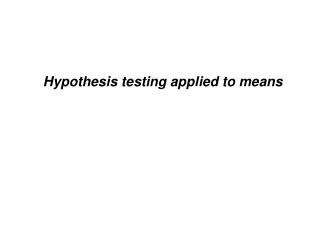DownloadDownload PresentationHypothesis testing applied to means

# Hypothesis testing applied to means

Download Presentation## Hypothesis testing applied to means

- - - - - - - - - - - - - - - - - - - - - - - - - - - E N D - - - - - - - - - - - - - - - - - - - - - - - - - - -
##### Presentation Transcript

1. Hypothesis testing applied to means

2. Characteristics of the Sampling Distribution of the mean The sampling distribution of means will have the same mean as the population :0 = :

3. Characteristics of the Sampling Distribution of the mean The sampling distribution of means has a smaller variance. s 2 s 2 s = s = x x N N s = standard deviation of the mean = standard error x This is because the means of samples are less likely to be extreme compared to individual scores.

4. Characteristics of the Sampling Distribution of the mean The shape of the sampling distribution approximates a normal curve if either: the population of individual cases is normally distributed the sample size being considered is 30 or more

5. X - m 115 - 100 z = = = 1 . 0 s 15 Probability is approximately .16

6. Example Jill now has to choose 25 intelligent people. 0 = 106 IQ test: := 100 F = 15. Hypotheses: H1: :1:2 H0: :1 = :2 = 100

7. Sampling Distribution :1 = :2 = 100 s 15 15 s = = = = 3 x N 25 5 X - m X - m 106 - 100 6 z = z = = = = 2 . 00 s s 3 3 x x Look up area in the tail of the curve = .0228 (one tailed) or .0456 (two tailed) If signficance level (") = .05, reject the Null Hypothesis

8. t Distribution • What happens if we do not have the population standard deviation? • We can use the sample standard deviation and an estimate. • Problem • Cannot use z and the normal distribution to estimate probability. • Sample variance tends to underestimate the population variance • Have to use a slightly different distribution - Student=s t Distribution X - m z = s x X - m t = s x

9. Degrees of freedom df = n - 1 Different t distribution for each degree of freedom

10. Sample: N = 56 0 = 104.3 S = 12.58 Norms: : = 100 Hypotheses: H1: :1:2 H0: :1 = :2 = 100

11. X - m X - m = t = s s x N 104 . 13 - 100 4 . 125 = = 12 . 58 1 . 682 56 t = 2 . 45 df = 56 - 1 = 55 tcrit or t.025 = " 2.009 tobs > tcrit Reject H0

12. Percentage Points of the t Distribution See Howell page 247

13. Matched-Sample t-test Use related samples In SPSS called the >Paired-Samples t-test' Data: Howell p 193

14. m = m - m = 0 H0: 1 2 D D - m D - 0 D - 0 t = = = s s s D D D N

15. D - 0 - 4 . 29 - 0 - 4 . 29 = = = - 1 . 49 s 16 . 04 2 . 88 D 31 N df = N - 1 (N is number of pairs of observations) df = 31 - 1 = 30 t.025(30) = " 2.042 tobs < tcrit Fail to reject H0

16. Two Independent Samples Distribution of Differences between Means

17. 2 2 s s 1 2 Variance of the Distributions of the Means & N N 1 2 s s Standard Error of the Distributions of the Means 1 2 & N N 1 2 2 2 s s Variance of the Distribution of Mean Difference 2 1 2 s + X - X = N N 1 2 1 2 2 2 s s Standard Error of the Distribution of Mean Difference 1 2 s = + X - X N N 1 2 1 2 m - m = 0 Mean of the Distribution of Mean Differences 1 2

18. t - test for two independent samples X - m t = s x ( X - X ) - ( m - m ) 1 2 1 2 t = s X - X 1 2 ( X - X ) - ( m - m ) 1 2 1 2 = 2 2 s s 1 2 + N N 1 2 ( X - X ) 1 2 = 2 2 s s 1 2 + N N 1 2

19. Pooled Variance estimate 2 2 ( N - 1 ) s + ( N - 1 ) s 2 1 1 2 2 s = p N + N - 2 1 2 ( X - X ) ( X - X ) 1 2 = 1 2 t = 2 2 s s 2 2 s s p p + 1 2 + N N N N 1 2 1 2 ( X - X ) 1 2 t = æ ö 1 1 2 s + ç ÷ p N N è ø 1 2 df = (N1 - 1) + (N2 - 1) = N1 + N2 -2 Degrees of Freedom: